### Category: Source code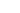## Another maze generator and solver in C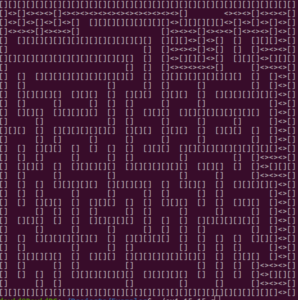I liked this one; it compiled perfectly without any changes and ran perfectly. It produces a maze of the specified size with a route. That’s not bad for a program written over 20 years ago. By developer Joe Wingbermuehle. You can view the source code here.

It runs in a terminal, just supply width and height characters like this. I compiled it into a file ex1.

`./ex1 15 15 s`

If you provide the s parameter, it will solve it as the screenshot shows using <> for the solved route. off for just the maze.## Another Minecraft game in C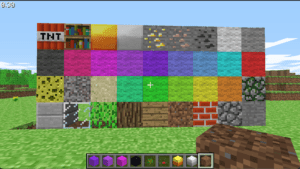If you remember back in November I mentioned a Minecraft server that was written in C.  Well now there’s another one that has appeared. Just lIke the other one it uses SDL2 and OpenGL and includes full source code.  This one uses clang.

It’s cross-platform for Windows and Mac and there are two different binaries, one for creative mode and one for survival mode.

It’s still a work in progress and needs sound effects and music, saving and loading levels and multiplayer to complete it. If you are learning C and want to see how a game like this is programmed, download the source code from GitHub and start studying it.## Portable Life in C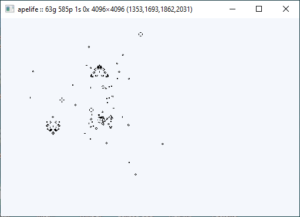I’ve mentioned Life before, this is the cellular automata as discovered by John Horton Conway. It’s perhaps less of a game and more of a recreation for anyone fascinated by programming. It’s hard to add up how many man-hours have been spent on it.

Developer Justine Tunney (aka Jart on Github) has developed a portable Life called Apelife. Portable as in it can run on Windows (graphically) and Linux (Text User Interface). The application is a modest 112 KB in size and is one of the fastest I’ve seen. When you have a large area and gliders whizz across it, you know its fast.

She is also the author of Cosmopolitan C which Apelife uses.  It lets gcc outputs portable binaries that will run on every Linux distro in addition to Mac OS X, Windows NT, FreeBSD, OpenBSD, and NetBSD. It’s rather ingenious.## Some Pretty fractals in C + SDL2

If you’ve ever wanted to draw fractals in C, here’s some code for you. These come from Misha on his(her?) GitHub page.  There are three as you can see. Each has their own program – barnsley.c, sierpinski.c and y-fractal.c. All three programs create an SDL2 window then display the image.

Note that this was coded for Linux. I modified the programs so they would run on Windows and the screenshot was done from my machine. I know these images are on the GitHub page.

If you want to compile and run, then on all three programs change the SDL include from

`#include <SDL2/SDL.h>`

to

`#include "SDL.h"`

Also with the barnsley.c you need to give initial values to mx and my in the renderFractals() function (lines 88 and 89) and change these two lines: (114 and 115). No other changes are needed to let them compile.

``````
mx_c = mx - win_w / 2;
my_c = my - win_h / 2;
```  to```
mx_c = mx - WIDTH / 2;
my_c = my - HEIGHT / 2;``````## DungeonRush – open source C + SDL 2 game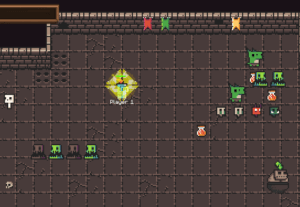I continue my quest, looking around for open source games in C that use SDL 2. The latest one is DungeonRush by developer Rapiz1 (does no one ever use their real names these days?) who hails from Wuhan.

It is not a rogue-like, but more a Snake-like game.   You move your hero around the playing area avoiding monsters and things fired at you while picking up stuff and people to give you extra lives.  As well as the difficulty levels it includes multiplayer mode as well though I haven’t tried that.

If you are learning C or games programming, it’s worth studying to see how others do things; this includes its own text drawing and high scoring and saving high scores.  It’s quite fun to play though I am rubbish playing it, even at the normal level. I’ve added this to the C Code links page. (Accessed on the top menu),

Snake games are interesting because if you use a circular buffer, no matter how big the snake grows, you can move it by just moving the head and tail elements. An O(1) operation!## Timing of SDL vs TTF fonts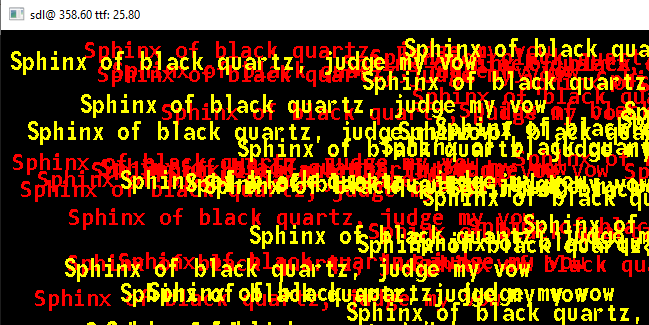So I got round to writing that SDL vs TTF timing comparison. However I had to make a few assumptions. The program was written for Windows but easily converts to Linux (apart from the timing code but I did that back in March for Linux) is about 250 lines long. I’ve uploaded it to GitHub, and the zip file contains not only the VS 2019 project and source but all the SDL2 dlls plus a free font MONOFONT.TTF (renamed to font.ttf) and test.png which is the bitmap font as a .png file.

Note you’ll have to edit the sdlttf.c file and change the paths to load the font .png and ttf files in lines 96 and 97.

I’d never used SDL_ttf before though it worked out easy enough. There are three levels of TTF – fast, not so fast and slow but pretty. I went for the fastest setting. The SDL text is in red and the TTF in yellow and are roughly the same height about 30 points.

If you read the caption you’ll see that my bitmap font drawing (I call it SDL) is nearly 14 x slower than TTF. In my code I prerendered the phrase “Sphinx of black quartz, judge my vow” which has all 26 letters a-z but is shorter than standard phrase “the lazy quick fox…”.

SDL_ttf renders the phrase into a surface which is a memory structure in RAM. Calling SDL_CreateTextureFromSurface (line 188) creates a texture from this (it copies the bitmap from RAM to VRAM) and so in my program I was just blitting this texture to the screen. The SDL printing in the print function (Line 157) gets each character, and calls printch (line 143) which works out where in the textFont texture that character and blits it. I’m guessing the overhead of blitting individual characters is what makes it so much slower.

So for fixed phrases where you can prerender the surface and texture, SDL_ttf is way faster and more flexible with colour and to a certain extent size. Colour is set at runtime while size is set when you prerender the font into the surface. If you want different sizes then you need multiple surfaces and corresponding textures for each size. Things like say a score would be a bit slower because it would need to be rendered each time and copied to a texture or prerender all 10 digits and have a texture for each and draw it much like the print method does.

The program is a little rough in places; it was thrown together over three nights.## Quicksort in C

Normally I wouldn’t mention a sorting algorithm, but by a lucky coincidence, when I had my interview before going to university (Queen’s University Belfast), the bloke who interviewed me back in 1977 was the inventor of Quicksort. Tony Hoare. He left very shortly after so I never got him as a lecturer,

As algorithms go, Quicksort is one of the faster. it works by splitting the list in two and then recursively sorting the two halves. The simplest sorting method Bubble sort uses a double loop and for very small numbers of items to sort is generally more efficient than Quicksort, but it doesn’t take long for Quicksort to catch up.

I was reminded of Quicksort by seeing this article and thought it would be interesting to do a series of test of sorting 5-50 items a few thousand times each in Quicksort and Bubblesort and see where the crossover point is. How many items is the minimum for Quicksort to become faster than Bubble sort?

If you take the Swap functions and BubbleSort from here and partition and quickSort functions from here and use my high precision timing code and add the code below then you can run the comparison. I did it twice, once in Debug and once in Release and the difference is much larger in release. I’ve zipped up the source code including the Visual C++ project code (It is C source code not C++ btw) and high performance timing code and put it on GitHub.

``````#define NUMLOOPS 5000

int main()
{
int startarr[]= { 86,91,50,5,79,64,2,57,26,63,12,61,12,92,40,38,11,
94,90,38,24,25,54,75,67,56,7,9,32,26,54,48,51,28,
90,50,37,53,8,75,30,25,59,57,92,42,25,33,84,46 };
int arr;
stopWatch stw;
double bTime, qTime;

bTime = 0.0;
qTime = 0.0;
for (int i = 3; i < 50; i++) {
printf("Bubble sort for %d = ", i);
for (int j = 1; j < NUMLOOPS; j++) {
memset(arr, 0, sizeof(arr));                     // Clear arr
memcpy(arr, startarr, sizeof(int) * i); // copy in i elements
startTimer(&stw);
bubbleSort(arr, i);
stopTimer(&stw);
bTime += getElapsedTime(&stw);
}
bTime /= NUMLOOPS;
printf("%12.10f.  ", bTime);
printf("Quicksort sort for %d = ", i);
for (int j = 1; j < NUMLOOPS; j++) {
memcpy(arr, startarr, sizeof(int) * i); // copy in i elements
startTimer(&stw);
quickSort(arr, 0, i - 1);
stopTimer(&stw);
qTime += getElapsedTime(&stw);
}
qTime /= NUMLOOPS;
printf("%12.10f\n", qTime);
}
return 0;
}``````

These are the first 15 and last 5 output lines in Release. The Bubble sort time for 7 is a bit of an oddity just for the actual numbers sorted. But its clear that by 11 values that Bubble sort is slower than QuickSort and the difference just gets worse up to 50. So up to 10 elements, Bubble Sort wins.

``````Bubble sort for 3 = 0.0000000358.  Quicksort sort for 3 = 0.0000000412
Bubble sort for 4 = 0.0000000407.  Quicksort sort for 4 = 0.0000000445
Bubble sort for 5 = 0.0000000474.  Quicksort sort for 5 = 0.0000000485
Bubble sort for 6 = 0.0000000677.  Quicksort sort for 6 = 0.0000000448
Bubble sort for 7 = 0.0000000191.  Quicksort sort for 7 = 0.0000000097
Bubble sort for 8 = 0.0000000405.  Quicksort sort for 8 = 0.0000000601
Bubble sort for 9 = 0.0000000510.  Quicksort sort for 9 = 0.0000000434
Bubble sort for 10 = 0.0000000568.  Quicksort sort for 10 = 0.0000000653
Bubble sort for 11 = 0.0000000633.  Quicksort sort for 11 = 0.0000000566
Bubble sort for 12 = 0.0000000702.  Quicksort sort for 12 = 0.0000000606
Bubble sort for 13 = 0.0000000845.  Quicksort sort for 13 = 0.0000000743
Bubble sort for 14 = 0.0000000931.  Quicksort sort for 14 = 0.0000001145
Bubble sort for 15 = 0.0000001040.  Quicksort sort for 15 = 0.0000000827
Bubble sort for 16 = 0.0000001142.  Quicksort sort for 16 = 0.0000000892
Bubble sort for 17 = 0.0000001448.  Quicksort sort for 17 = 0.0000000974
...
Bubble sort for 45 = 0.0000008478.  Quicksort sort for 45 = 0.0000002737
Bubble sort for 46 = 0.0000008561.  Quicksort sort for 46 = 0.0000002524
Bubble sort for 47 = 0.0000008948.  Quicksort sort for 47 = 0.0000002643
Bubble sort for 48 = 0.0000009421.  Quicksort sort for 48 = 0.0000003147
Bubble sort for 49 = 0.0000010042.  Quicksort sort for 49 = 0.0000002804``````## Can you compile this?

It’s just a little bit of C. I compiled it with these three compilers. MS VC (16.7.7 on Windows) gcc, and clang on Ubuntu 20.04 LTS. MS VC picked up the _Noreturn and complained, gcc 9.33 compiled it with not a whisper while clang 10.0.0 warned about void in the main function but compiled it anyway.

Both the clang and gcc compiled files ran and as you’d expect sat in an infinite loop until I control-c’d it.

However soon MS VC will compile it. According to this blog entry, MSVC from 16.8 preview 3 supports C11 and the _Noreturn feature (which tells the compiler that the function never returns) will be ok. Both gcc and clang support C11 so no problems.

``````#include <stdio.h>

_Noreturn void nrloop() {
while (1) {
;
}
}

void main() {
nrloop();
}``````

When would I use such a function, you might ask. IO can’t see me using it much unless I wnt to write a background thread function that just runs forever. I have indeed written such a function recently but it was in Delphi not C. Another use is a function that exits perhaps vi a jmp. It also lets the compiler know so it can optimize return code away.

As I said, I don’t think I’ll be using it much if at all.## So what do you think this code does?

Here’s a little C function. It’s not exactly intuitive what it does and I’ve renamed it to prevent giving it away.

``````  float mystery( float number )
{
long i;
float x2, y;
const float threehalfs = 1.5F;

x2 = number * 0.5F;
y  = number;
i  = * ( long * ) &y;
i  = 0x5f3759df - ( i >> 1 );
y  = * ( float * ) &i;
y  = y * ( threehalfs - ( x2 * y * y ) );
return y;
}``````

If it helps, this line is really the heart of it.

``    i  = 0x5f3759df - ( i >> 1 );  ``

Mystified? Well it’s a fast inverse square root. No one really knows who wrote it though John Carnack of Quake and Doom fame popularised it. There’s an interesting discussion about the author but no one really knows.

There’s less need for this type of thing nowadays because of all the fast silicon but back 20-25 years ago when graphics processors were in their very infancy, it was a highly useful optimization. Mind you , you’d need to know assembly language inside out to come up with stuff like this.## More on Shuffledness

So I’ve updated my shuffledness calculation. This is a bit of a work in progress so probably isn’t the last version.  One of the things I’m interested in is what is the optimal number of swaps to get a good shuffled deck of cards. The shuffle algorithm picks two random indexes in the array 0-51 and then swaps the contents.

Obviously doing this 10 times would only shuffle at most 20 cards and probably fewer as there’s no checks for repeats. The only check is that both indexes (indices?) are not the same. So I want to experiment and see what an optimal value for numTries should be..

``````void ShuffleDeck(int numtries) {
int firstIndex, secondIndex;
for (int i = 0; i < numtries; i++) {
do {
firstIndex = rand() % 52;
secondIndex = rand() % 52;
}
while (firstIndex == secondIndex);
int value = deck[firstIndex];
deck[firstIndex] = deck[secondIndex];
deck[secondIndex] = value;
}
}``````

Anyway, I’ve added a CalcDistance() function. This looks at each card and calculates how far it has moved from it’s original position.  It then divides this by the maximum distance it could have moved to get a move “factor”. These are summed up and divided by 52 to give an average move factor. That’s your CalcDistance() function.

``````float CalcDistance() {
float distance = 0.0f;
for (int i = 0; i < 52; i++) {
float distanceDiv = (i < 26) ? 51 - i : i-1;
//printf("%i: %3.0f\n", i, distanceDiv);
distance += (abs(deck[i] - i) / distanceDiv);
}
return distance/52.0f;
}``````

The only thing odd about this function is the distanceDiv value which starts at 51, decreases to 25 then increases to 50. The printf let me check that was correct.

This is the current state of the program which I’ve listed fully below. Enjoy! It’s 63 lines long.

``````// shuffledness.c : This measures how shuffled a deck of cards is
// cards are hjeld as values 0-51 in a 52 int aaray.

#include <time.h>
#include <stdio.h>
#include <stdlib.h>

int deck;
time_t t;

// Order the cards 0-51. 0 = Acew Hards,13 = Ace Clubs,26 = Ace of Diamonds and 51 = King of Spades
void InitDeck() {
for (int i = 0; i < 52; i++) {
deck[i] = i;
}
}

// Works on glovbal array deck and calculates a value for how displayed each card is
float CalcDisorder() {
int total=0;
for (int i = 1; i < 52; i++) {
total += abs(deck[i] - deck[i - 1]);
}
printf("Total = %d\n", total);
}

// Sum up distance card moved/max distance
float CalcDistance() {
float distance = 0.0f;
for (int i = 0; i < 52; i++) {
float distanceDiv = (i < 26) ? 51 - i : i-1;
//printf("%i: %3.0f\n", i, distanceDiv);
distance += (abs(deck[i] - i) / distanceDiv);
}
return distance/52.0f;
}

// Shuffle a deck by swapping two random cards a specified number of times
void ShuffleDeck(int numtries) {
int firstIndex, secondIndex;
for (int i = 0; i < numtries; i++) {
do {
firstIndex = rand() % 52;
secondIndex = rand() % 52;
}
while (firstIndex == secondIndex);
int value = deck[firstIndex];
deck[firstIndex] = deck[secondIndex];
deck[secondIndex] = value;
}
}

int main() {
/* Intializes random number generator */
srand((unsigned)time(&t));
for (int i = 0; i < 25; i++) {
InitDeck();
ShuffleDeck(1000);
printf("CalcDisorder() ==%f x %f = %f\n", CalcDisorder(),CalcDistance(),(double)CalcDisorder() * CalcDistance());
}
return 0;
}
``````

Because I’m multiplying the two factors (disorder and Distance, the final value is never getting much above 0.35 and I’d prefer it to be in the range 0-0.999. Suggestions welcomed.

.